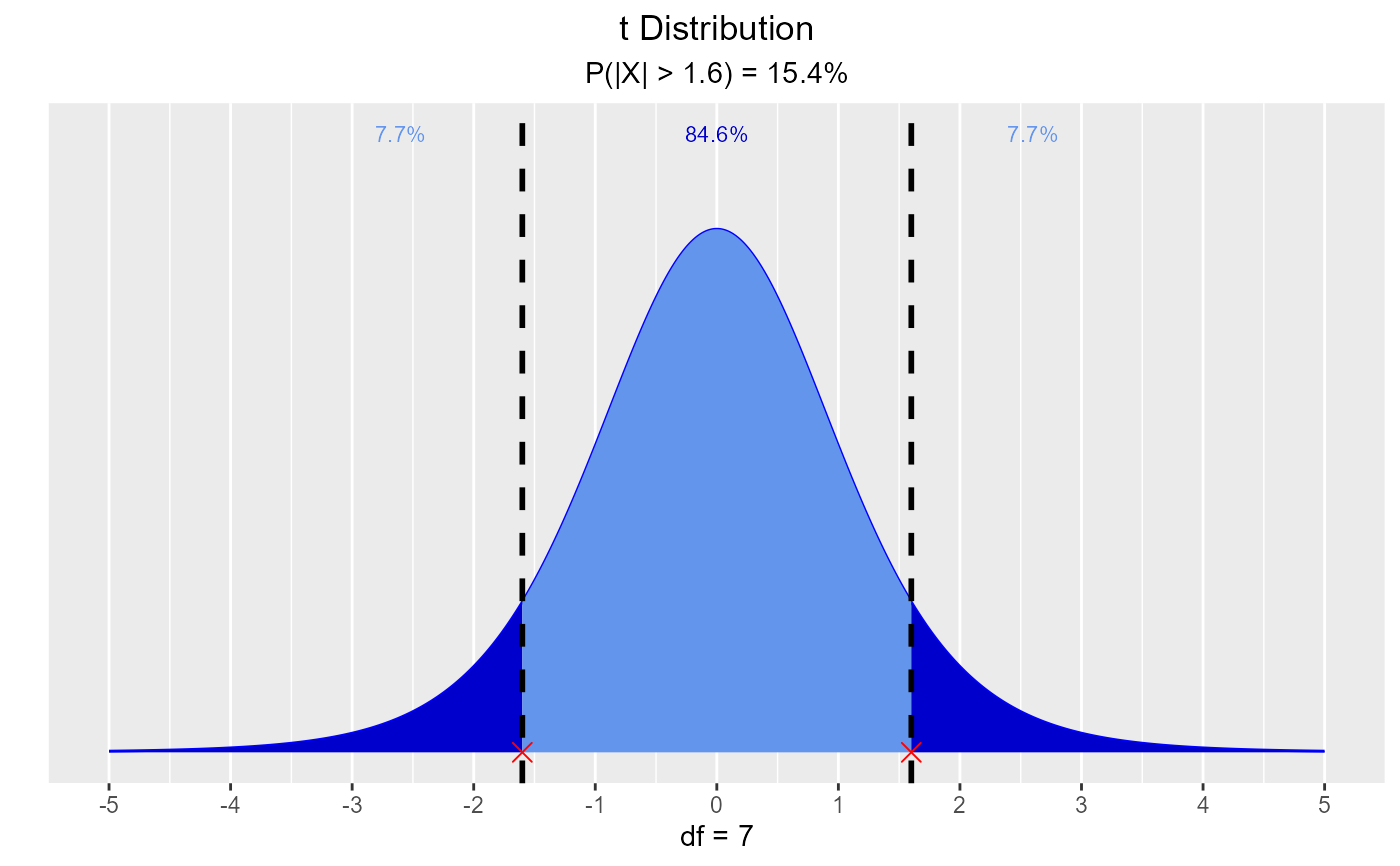Visualize how degrees of freedom affect the shape of t distribution, visualize quantiles out of given probability and probability from a given quantile.

vdist_t_plot(df = 3, print_plot = TRUE)

vdist_t_perc(
probs = 0.95,
df = 4,
type = c("lower", "upper", "both"),
print_plot = TRUE
)

vdist_t_prob(
perc = 1.6,
df = 7,
type = c("lower", "upper", "interval", "both"),
print_plot = TRUE
)

## Arguments

df Degrees of freedom. logical; if TRUE, prints the plot else returns a plot object. Probability value. Lower tail, upper tail, interval or both. Quantile value.

TDist

## Examples

# visualize t distribution
vdist_t_plot()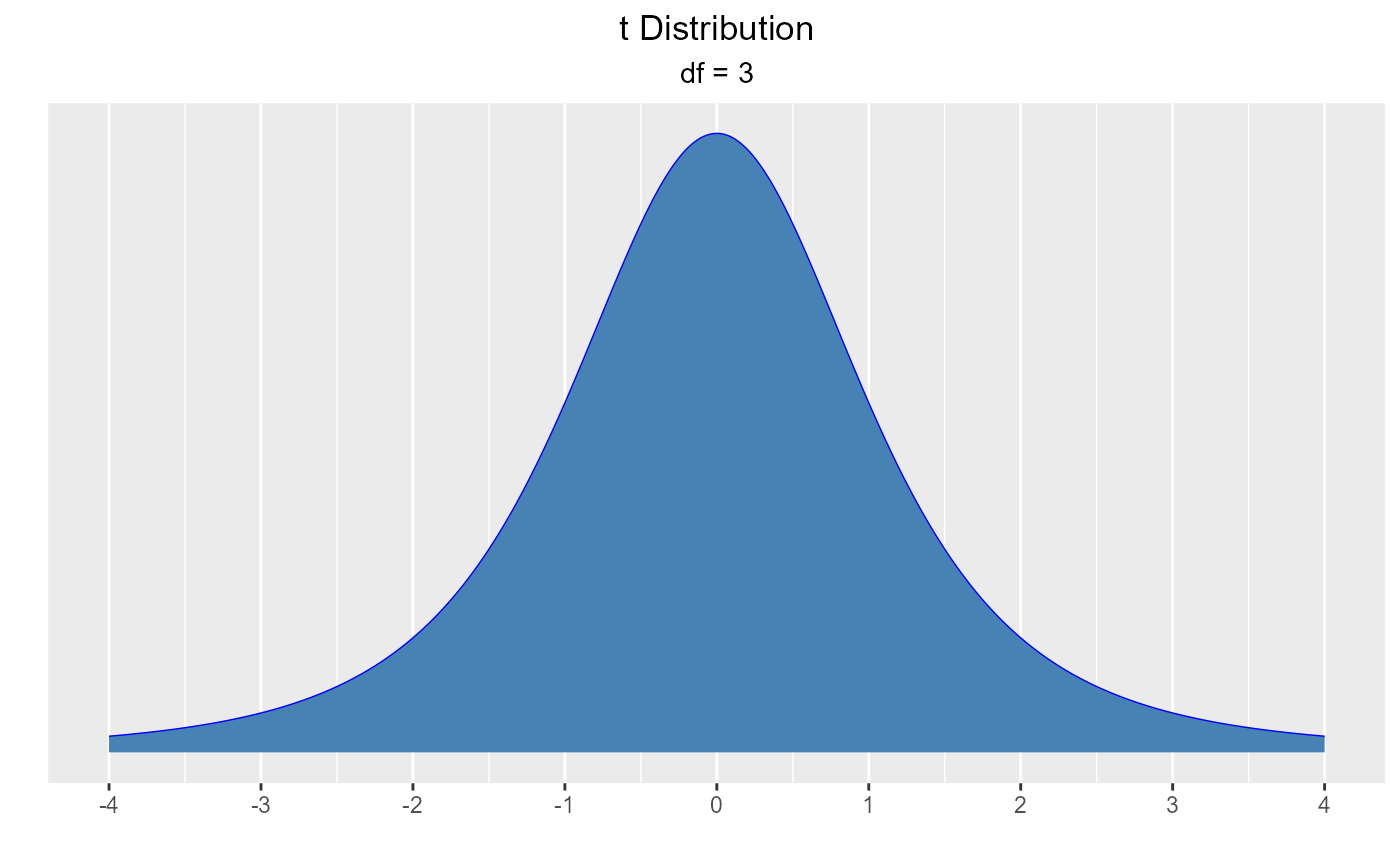vdist_t_plot(6)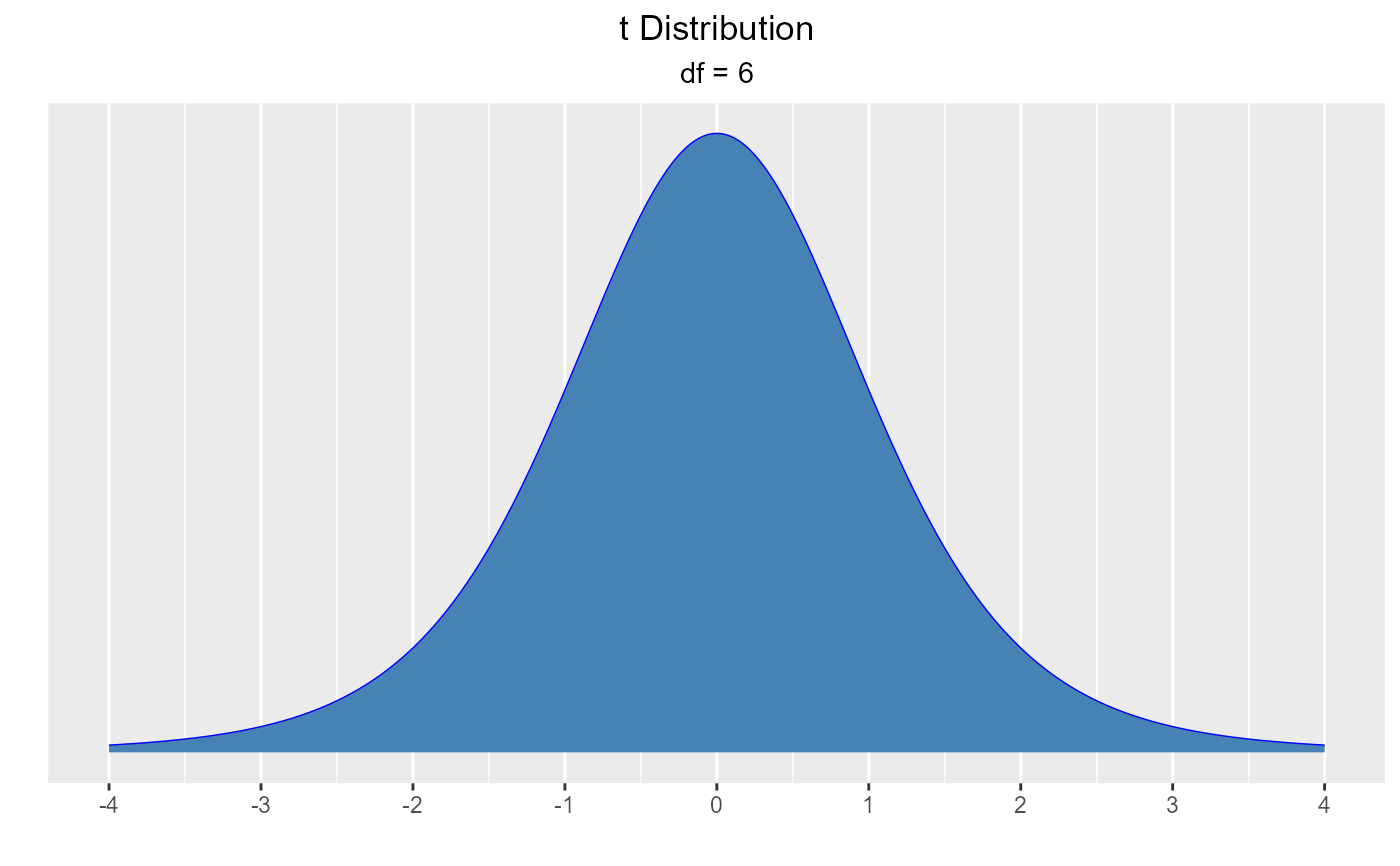vdist_t_plot(df = 8)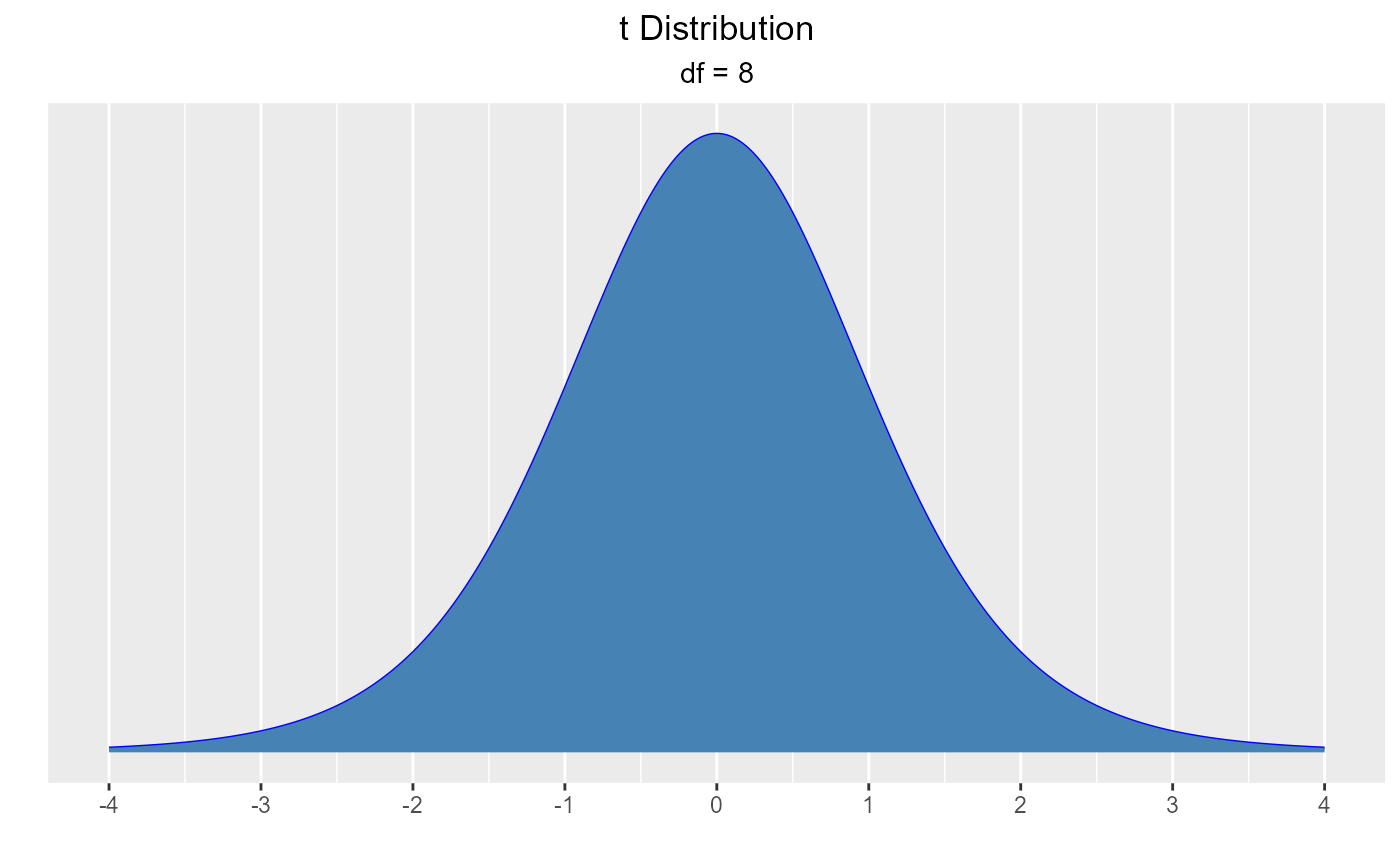# visualize quantiles out of given probability
vdist_t_perc(probs = 0.95, df = 4, type = 'lower')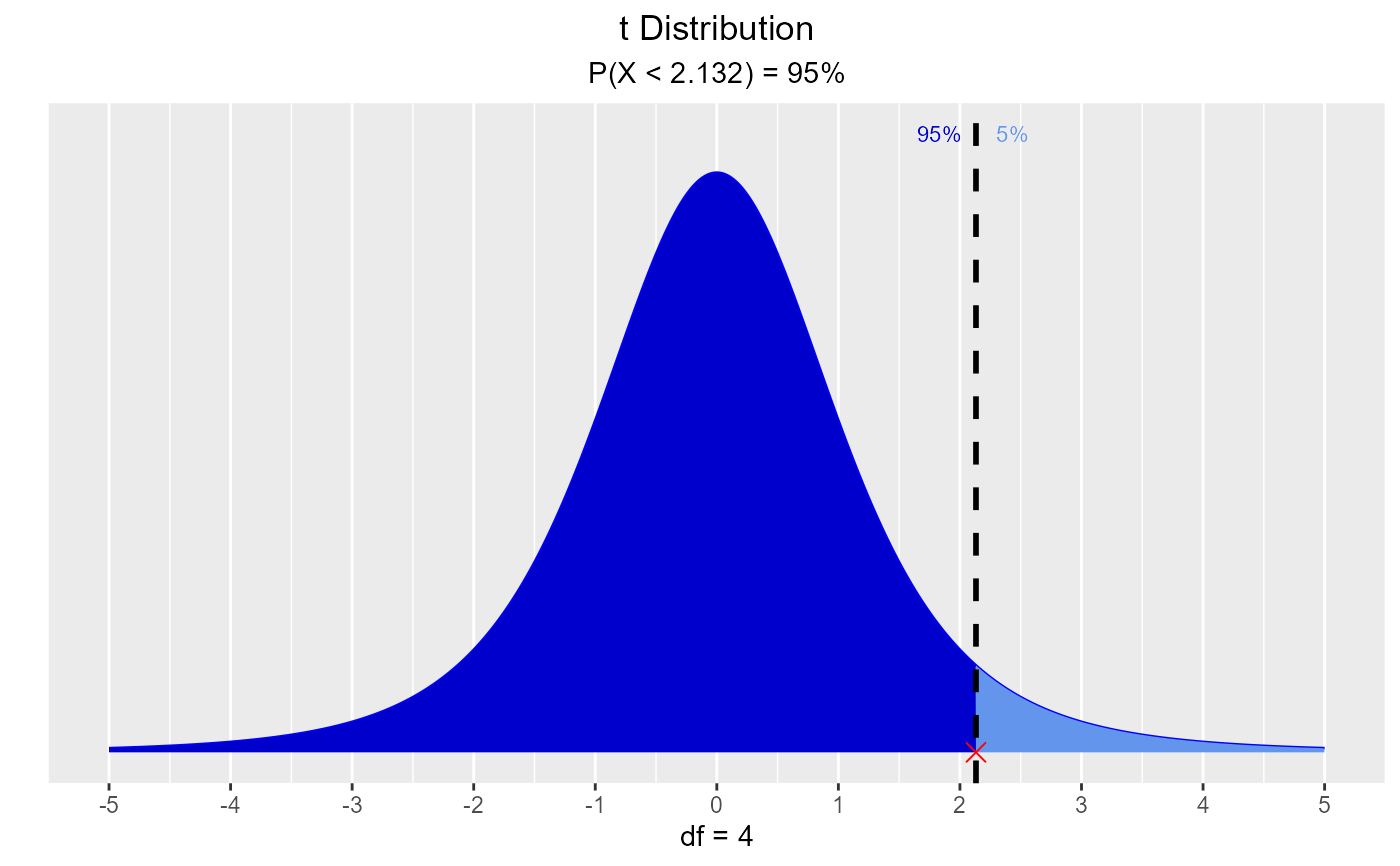vdist_t_perc(probs = 0.35, df = 4, type = 'upper')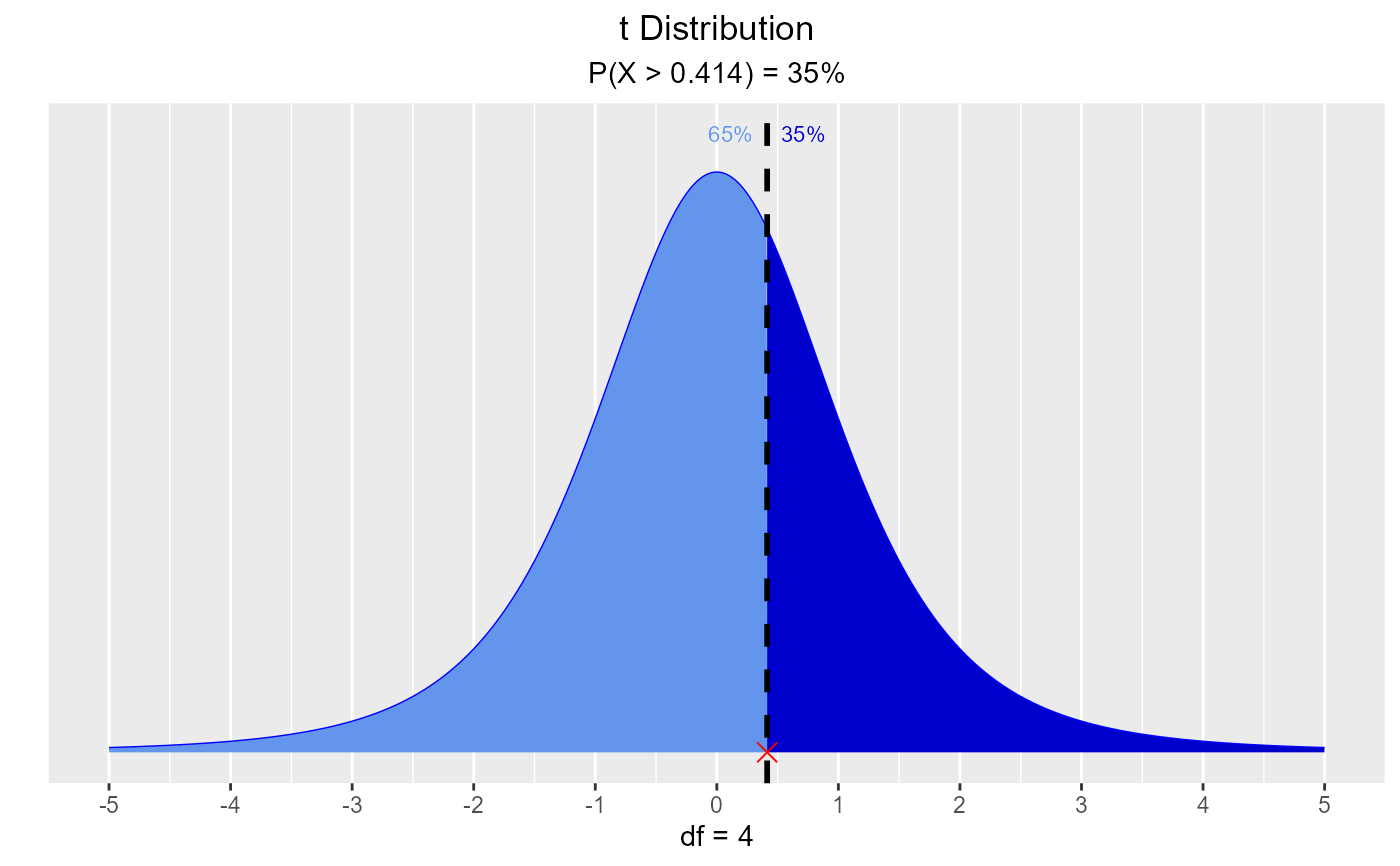vdist_t_perc(probs = 0.69, df = 7, type = 'both')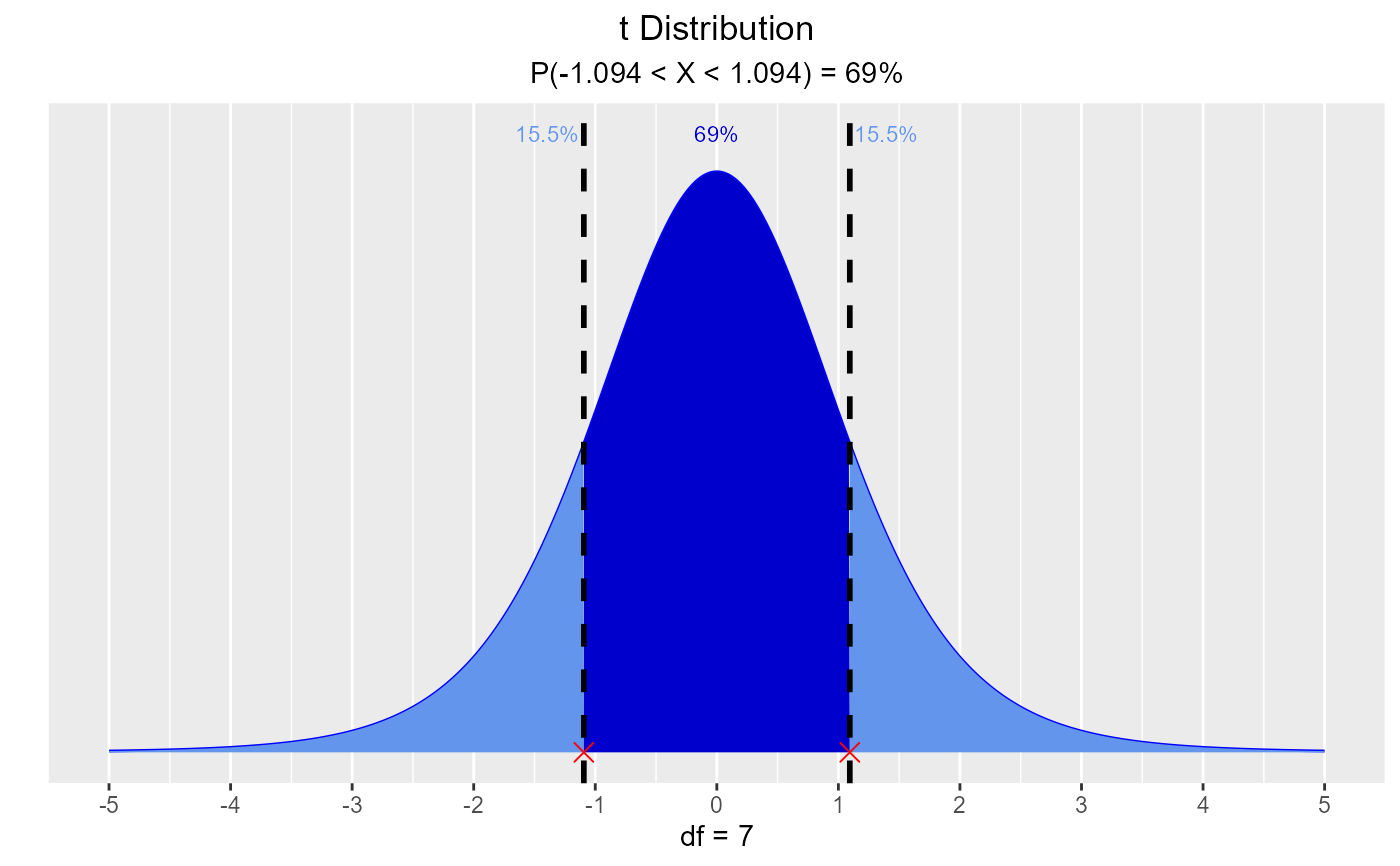# visualize probability from a given quantile
vdist_t_prob(2.045, 7, 'lower')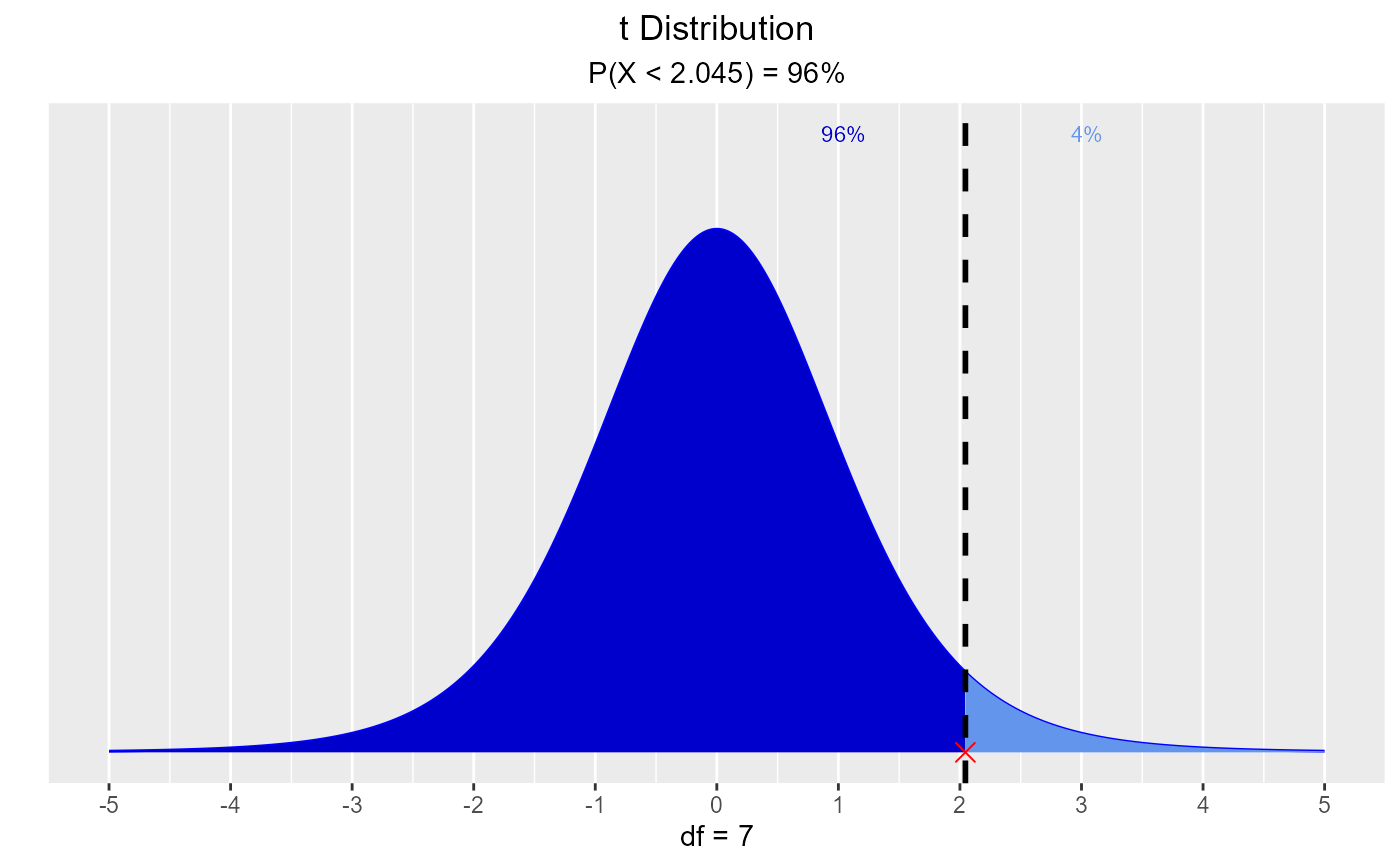vdist_t_prob(0.945, 7, 'upper')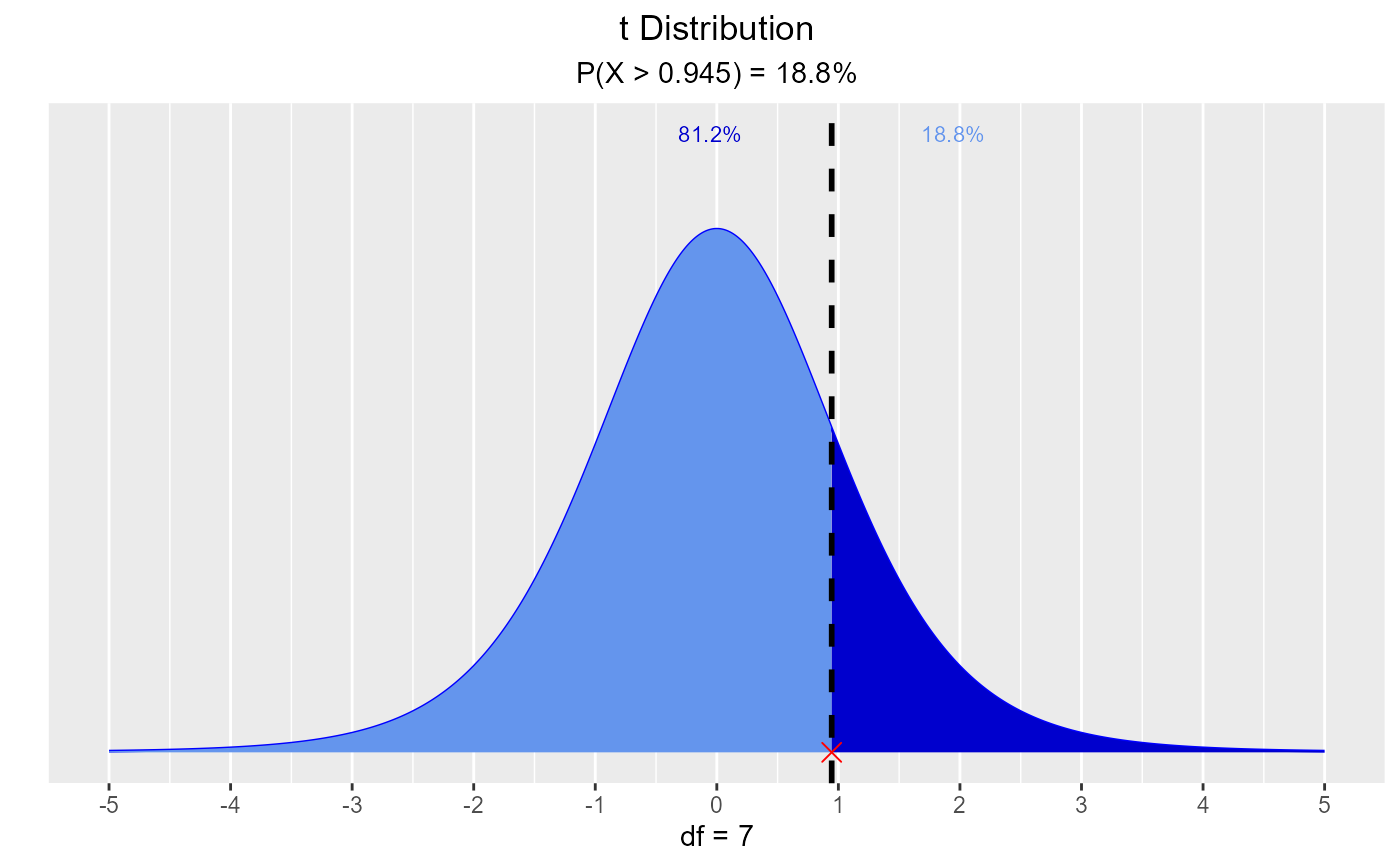vdist_t_prob(1.445, 7, 'interval')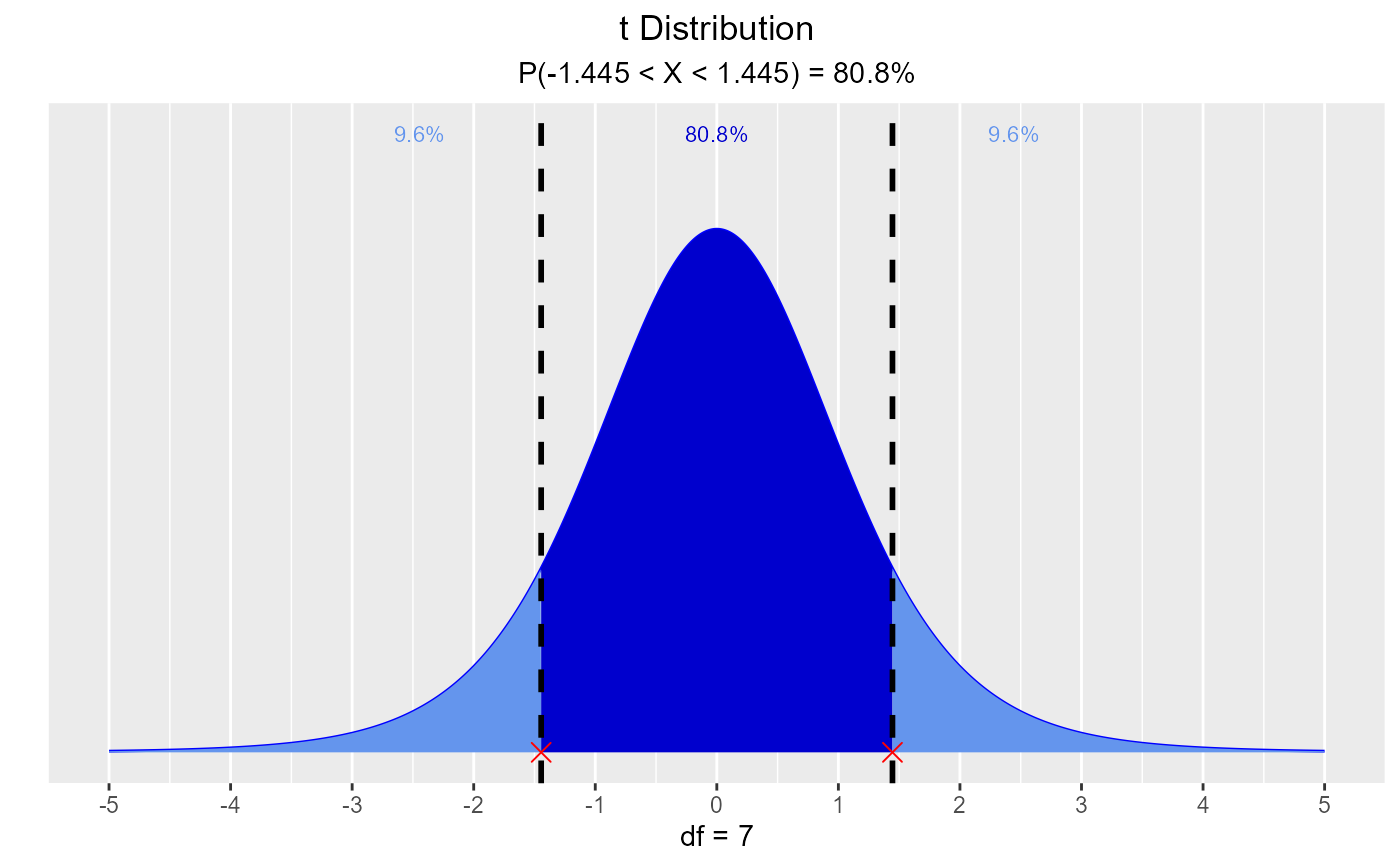vdist_t_prob(1.6, 7, 'both')